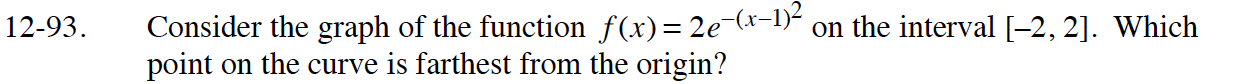### Home > CALC > Chapter 12 > Lesson 12.2.3 > Problem12-93

12-93.

Consider the graph of the function f(x) = 2e−(x−1)2 on the interval [−2, 2]. Which point on the curve is farthest from the origin? Homework Help ✎Any point on the function is (x, f(x)). Therefore the distance from the origin is: